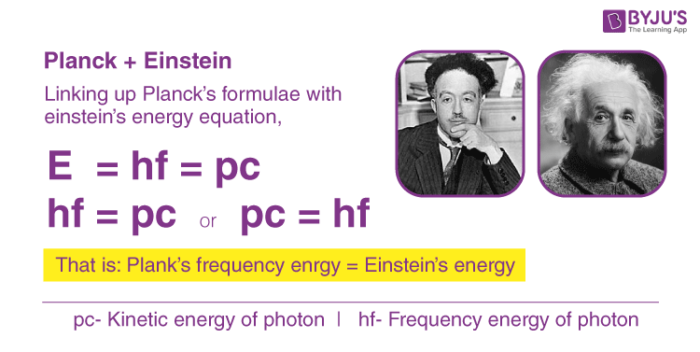Checkout JEE MAINS 2022 Question Paper Analysis : Checkout JEE MAINS 2022 Question Paper Analysis :

# De Broglie Relationship - Dual Behavior Of Matter

## De Broglie relationshipBohr’s model failed to explain many concepts related to the spectrum of different atoms and splitting of spectral lines in magnetic and electric fields. To overcome the shortcomings of Bohr’s atomic model, attempts were made to develop a more general model for atoms. One of those developments which contributed significantly in the formulation was the analysis of dual behaviour of matter. De Broglie proposed that as light exhibits both wave-like and particle-like properties, matter to exhibit wave-like and particle-like properties. This nature was described as dual behaviour of matter. On the basis of his observations, de Broglie derived a relationship between wavelength and momentum of matter. This relationship is known as the de Broglie relationship.

Considering the particle nature, Einstein equation is given as,

E= mc2 —- (1)

Where,

E= energy

m= mass

c = speed of light

Considering the wave nature, the Plank’s equation is given as,

E = hν      ——– (2)

Where,

E= energy

h = Plank’s constant

ν = frequency

From (1) and (2),

mc2 = hν ——– (3)

Frequency, ν can be expressed in terms of wavelength, λ as,

ν =

$$\begin{array}{l} \frac {c}{\lambda} \end{array}$$

For a general particle, c can be replaced with the velocity of object, v. Hence, equation (3) can be given as,

mv2 =

$$\begin{array}{l} \frac {hv}{\lambda} \end{array}$$

$$\begin{array}{l} \Rightarrow \lambda~=~\frac{h}{mv} \end{array}$$

The above equation is known as de Broglie relationship and the wavelength, λ  is known as de Broglie wavelength. Diffraction of electron beams explains the de Broglie relationship as diffraction is the property of waves. An electron microscope is a common instrument illustrating this fact. Thus, every object in motion has a wavelike character. Due to a large mass, the wavelengths associated with ordinary objects are so short that their wave properties cannot be detected. On the other hand, the wavelengths associated with electrons and other subatomic particles can be detected experimentally.